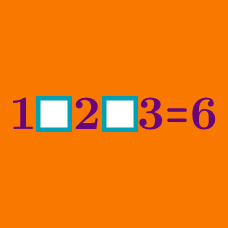Logic

# Operator Search With Parenthesis

$2 \; \square \; 2 \; \square \; 2 \; \square \; 2 \; \square \; 2$

Fill in the blanks above with the mathematical operators $$+ , - , \times , \div$$.

What is the smallest possible resultant number?

 Note:

• You don't have to use all 4 mathematical operators.
• The answer can be a negative number.

$5 \; \square \; 4 \; \square \; 3$

Using the operations of $$+, -, \times, \div$$ and parenthesis as many times as you like, which of the following $$\color{red} { \text{cannot} }$$ be a resultant number?

$1 \; \square \; 2 \; \square \; 3$

Using the operations of $$+, -, \times, \div$$ and parenthesis as many times as you like, is it possible to obtain a result of 4?

$3 \; \square \; 3 \; \square \; 3 \; \square \; 3 \; \square \; 3$

Fill in the blanks above with the mathematical operators $$+ , - , \times , \div$$.

What is the largest possible resultant number?

 Note: You don't have to use all 4 of those mathematical operators.

$4 \; \square \; 3 \; \square \; 2$

Using the operations of $$+, -, \times, \div$$ and parenthesis as many times as you like, is it possible to obtain a result of 10?

×